# 代数拓扑简介（下）：同调论

/// 注：本文仅提供对同调相关概念的直观理解，不包含严谨的推导内容，文中若有错误欢迎指正。### 链与链群1. 计算下图阴影区域二维链的边缘链，这条链的方向已经标注出来了。我们还是按三角形的顺序来记录链的方向。于是要求的边缘链为$$\partial (ade+aeb)=\partial(ade) + \partial(aeb) = (ad + de + ea) + (ae + eb + ba)$$注意$ea = -ae$，所以有$\partial (ade+aeb)= ad + de + eb + ba$，边缘为下图所示。任何图形的边界都是封闭的，换句话说边缘的边缘一定是0，即对任何链$A$都有$\partial\partial A=0$，读者可以自行验证上图红色的链确实边界为0。
2. 下面这个二维链的边缘链又是什么呢？读者可以自己计算一下，答案放在下一个小结说。### 闭链群与边缘链群### 同调类与同调群

1. 之前说，在同一个连通分支内的各个重数一样的单点都是同调的，要对所有的零维链按照同调等价类分类，只需要统计每个连通分支中点的总数目（重数）即可。比如一个有三个连通分支的图形的每个分支中所有点等价，我们就可以合并同类项，所有零维链肯定能写成$ax+by+cz$的形式，这些零维同调链连同加法运算就叫零维同调群，它们之间的加法也就是$x,y,z$前面的系数对应相加，这跟三维空间中的整数($\mathbb{Z}$)坐标点之间的加法一样，我们记作$\mathbb{Z}^3$。我们不难拓展到任意有$i$各连通分支的拓扑空间，它的零维同调群是$\mathbb{Z}^i$，即同构于$i$维空间整数坐标的加法群。这里提醒一下链群与同调群之间的关系：链群是单纯的所有链之间的加法运算，不管是不是闭合的图形统统都在里面，且图形改变后各个链也会变化；而同调群是闭链群中构造的同调等价类之间的加法运算，它反映图形的拓扑信息。试想如果直接在零维链群上定义加法，那么图形中有多少个点就有多少自由度，这个数量就太多了，对数连通分支个数完全没帮助。
2. 下面来看一维链的情况。为了方便计算边缘链，我们把下面左图的图形做三角剖分为右图。这样通过三角剖分计算的同调群，称为单纯同调，因为三角剖分继续推广到三维是四面体，四维是五胞体，它们统称$n$维单纯形。单纯同调的好处是，它可以很清晰地把高维图形的方向说清楚。首先要找到所有的闭链。不妨直接硬算：列举出所有的链，图形有十二条边，链就有十二个自由度，这里设了十二个变量$x_1$至$x_{12}$，令它的边缘链为0，可以得到一个方程：
$\partial (x_1 ab+x_2 bc+x_3 ca+ x_4 de$ $+ x_5 ef + x_6 fd+ x_7 ad + x_8 be$ $+ x_9 cf + x_{10}ae + x_{11}bf+x_{12}cd)$
$=(x_1-x_3+x_7+x_{10})a$ $+(x_2-x_1+x_8+x_{11})b$ $+(x_3-x_2+x_9+x_{12})c$ $+(x_4-x_6-x_7-x_{12})d$ $+(x_5-x_4-x_8-x_{10})e$ $+(x_6-x_5-x_9-x_{11})f=0$
没有边界意味着以上合并同类项后的每项系数均为零，即闭链的系数需要满足下面方程组：
$$\begin{cases} x_1-x_3+x_7+x_{10} = 0 \\ x_2-x_1+x_8+x_{11} = 0 \\ x_3-x_2+x_9+x_{12} = 0 \\ x_4-x_6-x_7-x_{12} = 0 \\ x_5-x_4-x_8-x_{10} = 0 \\ x_6-x_5-x_9-x_{11} = 0 \end{cases}$$
十二个变量六个方程，看似闭链还剩6个自由度，但学过线性代数的话不难验证这个方程的系数矩阵的秩为5，即有一个冗余的方程，闭链其实还有7个自由度。所有的闭链都可以由以下7个闭链叠加“生成”得到。
我们继续看看边缘链：所有的二维链都由六块边缘不同的三角形组成，自然边缘链的自由度为6。
下图中画出了闭链、边缘链的“生成元”：其它的闭链、边缘链可以由它们通过加法与数乘运算组合出来，比如这里画出的所有的链都是顺时针的，而逆时针可以由顺时针乘以-1得到。我们再看看同7个闭链中有六个都是边缘链它们都同调于0链，只有剩下一个不是，因此一阶同调群为剩下这个圈生成的加法群，即自己跟自己叠加，只有一个自由度，同构于整数加法群$Z$，我们看到空心圆盘的同调群跟同伦基本群是一样的。现在我们再来看看前面思考题要求的边缘链：直观上看内外两圈道路如果方向相反它们是可以扫过环状的实心区域形变收缩然后对消，但单独一个圈却不是任何图形的边界，永远也消不掉。从这个例子不难看出，给一个只要能三角剖分的很复杂的拓扑空间，都可以把一个连续的几何问题变成离散的代数问题，具体则是线性代数跟矩阵秩相关的算法，可以交给计算机处理，因此理论上任何空间的同调群的计算都没有任何技术困难。
3. 再来看看球面的同调群，它是连通的，只有一个连通分支，因此零维同调群为$\mathbb{Z}$，它上面的所有圈都是可缩的，自然也找不到不同调于0的一维链，这表明球面没有任何一维孔，一维同调群为0。球面有二维孔吗？整个球面其实就是唯一的封闭2维面，它很明显是三维实心球体的边界，但这里我们考虑的只是二维球面本身，不存在任何3维的图形，那么它自然就不是边缘链，因此球面的二维同调群就是整个球面叠加生成的，只有一个自由度，因此也是$\mathbb{Z}$。这里我们发现，拓扑空间的各阶同调群往往都跟$n$维空间中的整数坐标的加法群一样，这些空间的维度就是同调群的自由度，这样也就抓住了孔的个数。比如球面的各阶同调群分别为$\mathbb{Z},0,\mathbb{Z}$，则说明它有一个连通分支，没有一维洞，还一个球面形的二维洞。我们将这些$i$维同伦群的自由度记作拓扑空间的Betti数$b_i$，对于球面有$b_0=1, b_1=0, b_2=1$，它反映了各维度的洞的个数。
4. 计算环面的同调群。整个环面是连通的，所以$b_0=1$。要求$b_1$的话我们着重关注这些经纬线，看看它们是否是某二维图形的边界。沿经线切开，剩下图形的边界是两个圆圈，要保证方向一致的话，它们的方向必须相反。当两个圆周粘回去时，恰好抵消，得到没边界的整个曲面，也就是说单独的经线确实不是任何二维图形的边界，它就是我们要找的一个非零调的链。同理纬线圈也一样，算上这些圈的组合，可以证明所有的同调链都能写成$a$经线$+b$纬线的形式，它们之间的加法运算跟二维向量之间的加法是一样的，一共有两个自由度，说明圆环有两个一维孔，Betti数$b_1=2$。最后跟球相似，圆环面本身有个中空的实心环孔结构，由于我们没有考虑圆环内部的三维空间，所以整个二维环面只是一层套在环孔上的“壳”，它不是零调的。故$b_2=1$。因此圆环面所有同调群的维数（即Betti数）分别为：$b_0=1, b_1=2, b_2=1$。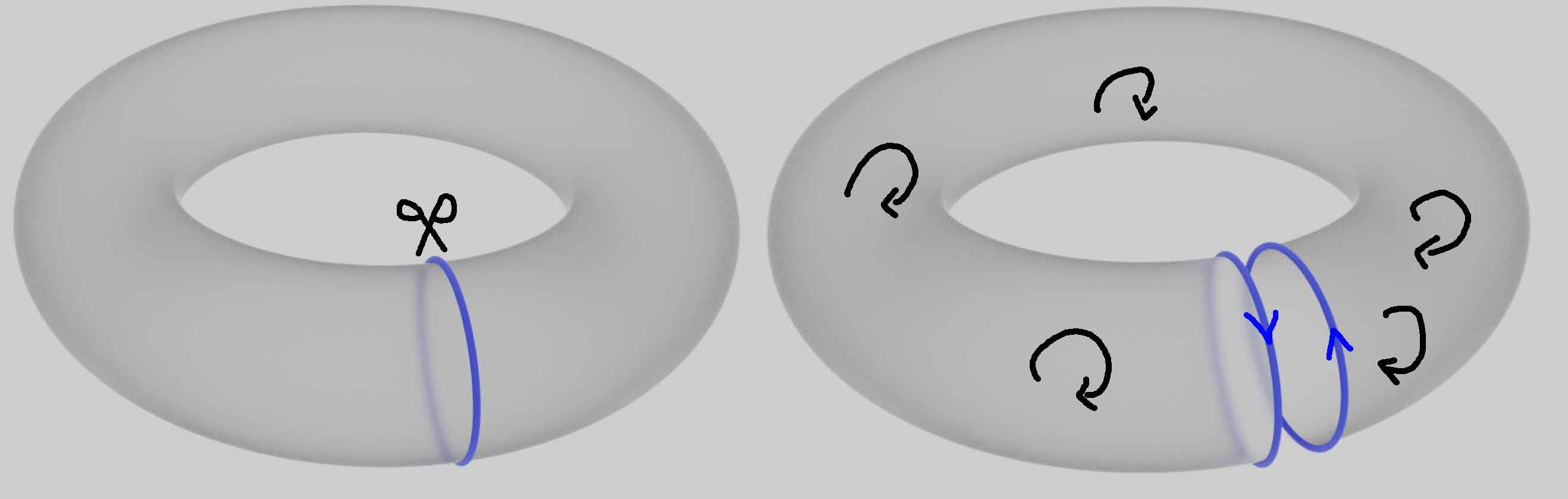5. 圆环内部的三维实心区域的同调群又是什么样的呢？首先这个二维环面现在已是实心圆环体空间的边界，因此它是零调的，没有二维孔，即$b_2=0$;其次，圆环上的经线圈现在也是可缩的了，沿经线圈把环体砍开得到的截面的边界就是经线本身，说明经线是同调于0的边缘链，但纬线仍然不可缩，只剩一个自由度，所以$b_1=1$。它还是一个连通图形，故$b_0=1$。总结一下圆环内部的三维实心区域的Betti数分别为：$b_0=1, b_1=1, b_2=0$。我们可以看到，圆周是它的形变收缩核，实心圆环与一维的圆周同伦，所以它的各阶同调群跟一维的圆周是等价的。
6. “8”字形的同调群：图形连通，$b_0=1$；分别绕两个圈的链都不是边界链，一维同调群为$\mathbb{Z}^2$，$b_0=2$。注意之前计算过“8”字形和环面的同伦基本群，它们分别是自由群$\mathbb{Z}*\mathbb{Z}$和交换群$\mathbb{Z}^2$。由于同调群的可交换性，它们之前的区别被抹杀掉了。可以证明任意拓扑空间的同伦基本群添加交换律后就能得到它的一阶同调群。
7. 超立方体的零维骨架：16个孤立的顶点：$b_0=16$，大于等于1的Betti数均为0。
8. 超立方体的一维骨架：32条棱构成的一张连通的网，显然$b_0=1$。我们可以通过同伦变换把立体的超立方体的洞给数出来共17个，即$b_1=17$：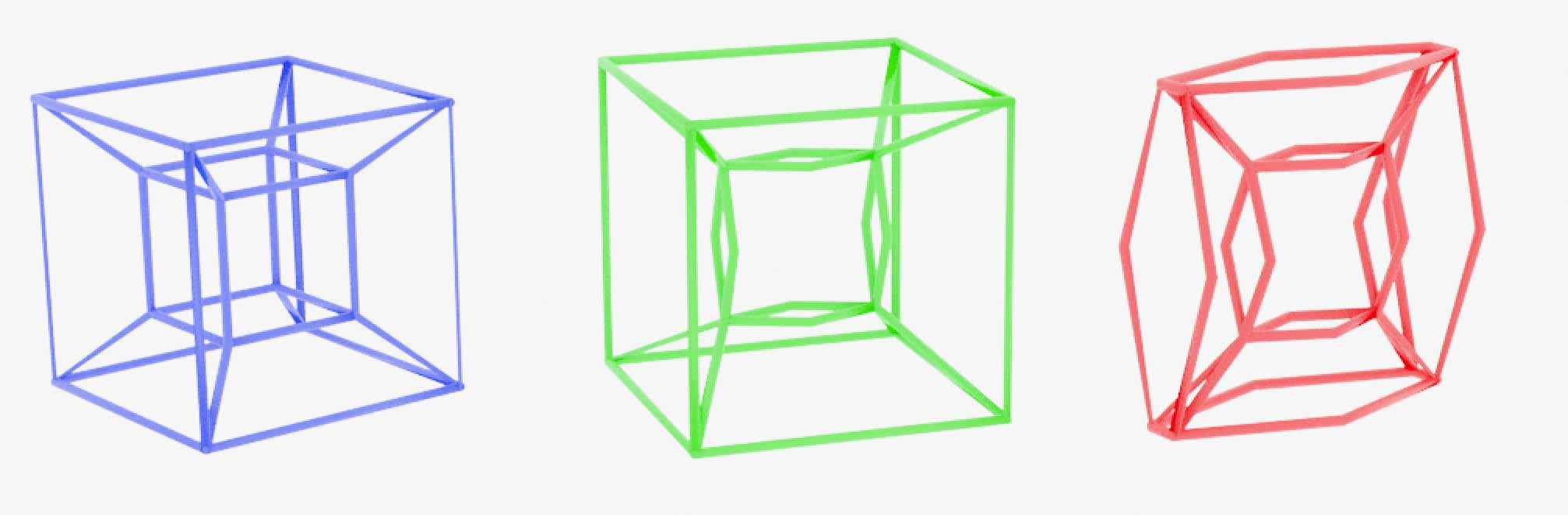其实还有个巧妙的方法计算任意图（Graph）的一维环孔数$b_1$。我们先随便构造一颗树，即没有环路的的图来覆盖所有顶点，其它没被树覆盖的边的数量就是一维环孔的数量。因为没环路的树是可缩成一点的，这样其它没被树覆盖的边就变成了圆周，整个图形就是那些圆周的单点并。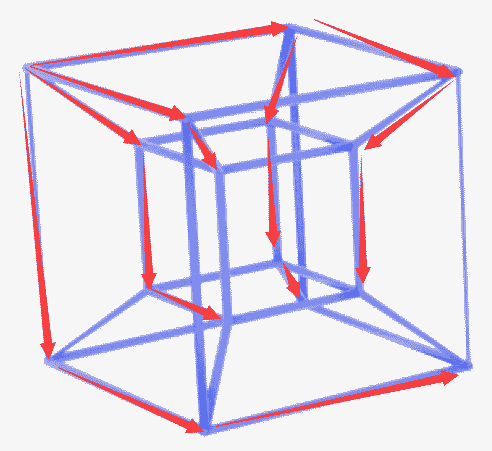9. 超立方体的二维骨架：24个正方形面把三维空间分成了互不连通7块胞腔。注意我们数超立方体的八个胞腔还要数最外面的大立方体胞，但同调的意义下一个包住大立方体胞的二维链可以由分别包住里面七个胞腔的七条二维链相加得到，所以独立的二维孔洞只有7个，即$b_2=7$。这个图形与实心立方体内有七个气泡的图形同伦，气泡无法拴住一维的圈，即所有一维的闭路径可缩，因此有$b_0=1,b_1=0,b_2=7$。
10. $n$维球面$S^n$上小于$n$维的球面都是可缩的，因此有 $b_0=1, b_1=b_2=..b_{n-1}=0, b_n=1$。
11. 根据同伦一定同调，$n$维空间$\mathbb{R}^n$、实心球$D^n$均与单点同伦，所以Betti数为$b_0=1, b_1=b_2=..b_n=0$。
12. 有时候同调群并不能区分不同伦的图形。比如以下左边图形（两圆周和一个球面的单点并）跟右边环面，它们都是1个连通分支、2个一维孔、一个二维孔，$b_0=1, b_1=2, b_2=1$。后面我们将用新的上同调论来区分它们。13. 下面我们来看看球环与环球的同调群：球环的纬线圈不可缩，与纬线垂直的是经球面，所有的经线都能在经球面上缩成一点，但二维的经球面本身不可缩，于是有$b_0=1, b_1=1, b_2=1, b_3=1$。其实环球与球环同胚，它们互相互换经纬线，即环球的有一维的不可缩经线圈与二维球面形的不可缩纬面，因此同调群一样。

### 欧拉公式的推广：欧拉-庞加莱公式

$\chi=C_0-C_1+C_2-C_3+….C_n$$=b_0-b_1+b_2-b_3+….b_n。 这个公式看似证明很难，但k维“面”数C_k、各维Betti数其实都是一些群的自由度，我们只要理清它们之间的关系就好。对于三角剖分来说，顶点C_0的数目正是三角剖分上的所有零维链群的自由度，同理边的数目C_1是一维链群的自由度，面的数目C_2是二维链群的自由度。我们再看看同调群的自由度是怎么来的：同调群是在所有的闭链群中，将边缘链视为等价类后剩下的商群，记i维边缘链群自由度为B_i，i维闭链群自由度为Z_i，则有z_i=b_i+B_i。闭链群与一般链群之间是什么关系呢？如果我们将封闭的链视为“0”，构造一种新的等价类，那么在这个等价类意义下非零的链都是不封闭(即有边界的)的图形，且拥有相同边界的两条链相差一个封闭链，所以有多少不同的边缘链就有多少个等价类，即C_i=B_{i-1}+Z_i，两式合并一下有C_i=b_i+B_{i-1}+B_i。把这些东西全都代入到要证的等式左边，注意所有0维链都是闭链，即第一项C_0=Z_0=b_0+B_0有： C_0-C_1+C_2-….=$$(b_0+B_0)-(b_1+B_0+B_1)+(b_2+B_1+B_2)-….$

### 不可定向（单侧）曲面的同调群1. 如果我们强行在矩形内画出定向一致的二维链，则矩形的边缘无法抵消，反而会变成两倍，所以这个二维链有边界，不是闭链。2. 如果我们从下图左上角的那个三角形出发往右走逐渐添加相同定向的三角形，注意越过矩形边缘时根据矩形两边的连接关系定向会反向，最后我们得到的二维链还是有边界的。## 上同调论(Cohomology)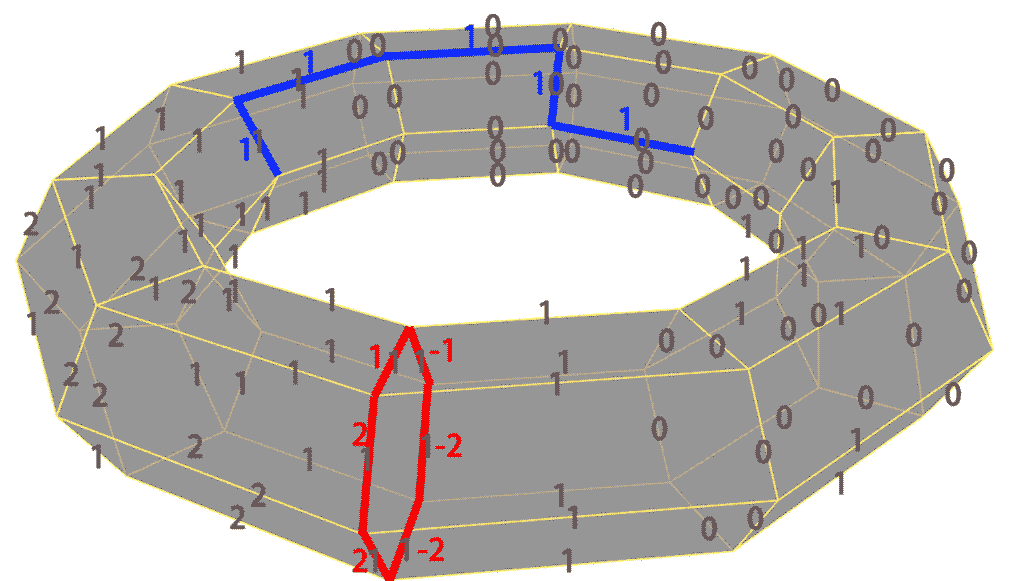### 上边缘算子### 上闭链### 上边缘链给定一条$n$维上链，怎么判断它是否为上边缘链呢？或许我们能够把那个$n-1$维上链找出来：还是以刚才上图中的一维上链为例：设零维链在顶点$a$处的值为$x$，又因为$\partial (ad) = a-d$，且$ad$上标的数字为$1$，所以我们能够得到零维链在顶点$d$处的值为$x-1$，这样通过一条边一条边的计算最终我们就能构造出0维上链在所有顶点上的值。然而，如果通过不同路径推出零维上链在同一点的值不一样的话就矛盾了，这时我们就找不到符合要求的零维上链，从而该一维上链就不是上边缘链。我们发现一条一维的上链是不是上边缘链的关键在于它是否存在一种“路径无关性”，这种路径无关性可以等价于同起点同终点的两条不闭合的下链之差要被上链映射到0，这些下链一定是闭合的，因此上边缘链一定要把所有闭合的下链映射为0来保证路径无关性。怎样证明这个结论呢？$n$维的闭合下链是没有边缘的，如果$n$维上链不将它映射为$0$，$n-1$维上链会把闭合下链的边缘映射到这个非零值，这跟闭合下链没有边缘是矛盾的。

### 上同调### 德拉姆上同调简介

$$\nabla E = 0$$

$$\nabla \phi = E$$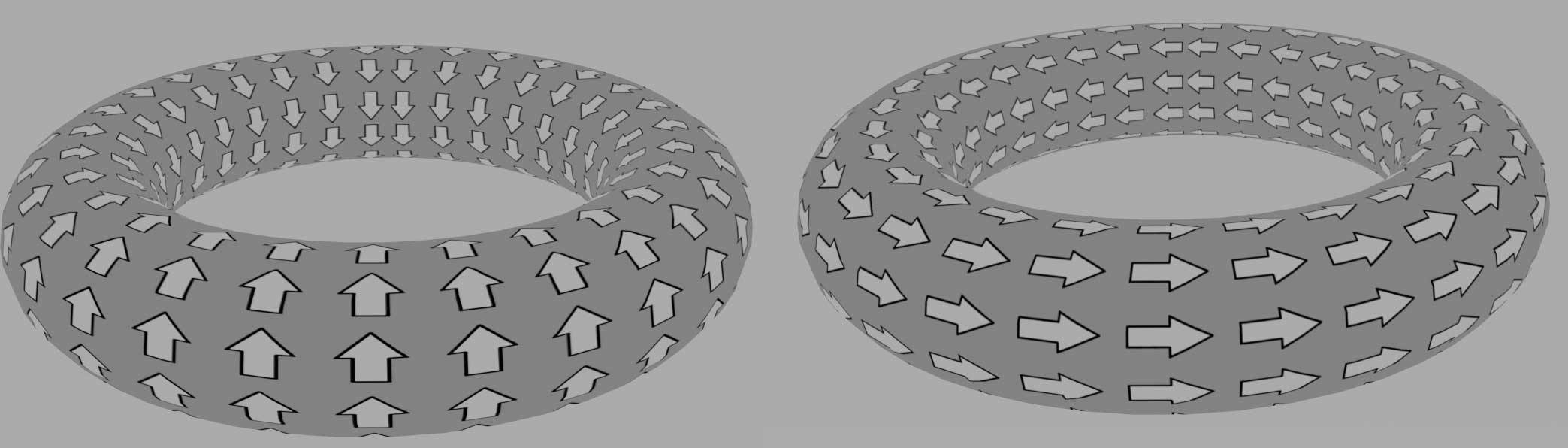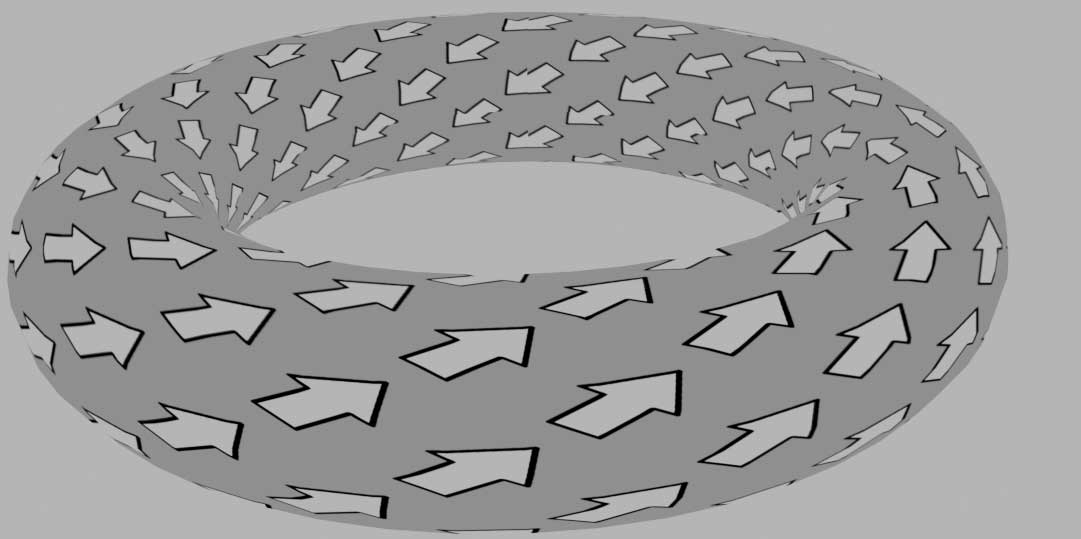### 上同调环### 庞加莱对偶性